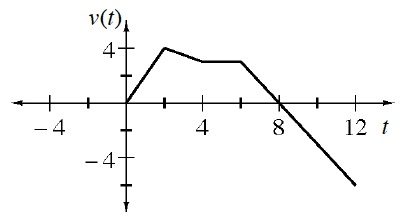### Home > APCALC > Chapter 2 > Lesson 2.1.1 > Problem2-13

2-13.

A bug is walking on your graph paper along the $x$-axis. The bug’s velocity (in feet per second) is shown on the graph at right.

1. When did the bug turn around?

Recall that positive velocity represents moving forward (away from the starting point) and negative velocity represents moving backwards (towards the starting point).

2. When was the bug’s speed the greatest?

Speed is the absolute value of velocity. All negative velocities become positive.

3. After $12$ seconds, how far is the bug from its starting position?

Distance from the starting point (i.e. displacement) represents the accumulated distance traveled. This bug moved both forward and backwards. Be sure to consider both positive and negative travel.

Distance from the starting point (i.e. displacement) can be represent by the area under a velocity curve. Compute this area using geometry. Recall that negative area means the bug is moving backwards.

4. Remember that acceleration is the rate of change of velocity. Calculate the acceleration of the bug at the following times.

1. $1$ second

1. $5$ seconds

1. $10$ seconds

$\text{rate of change of velocity }= \frac{\text{velocity}}{\text{time}}= \text{slope}$